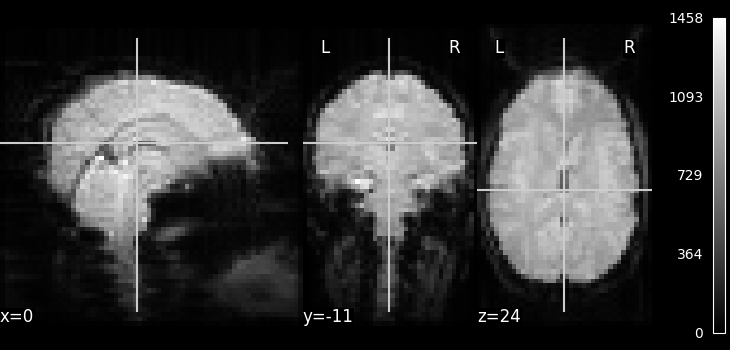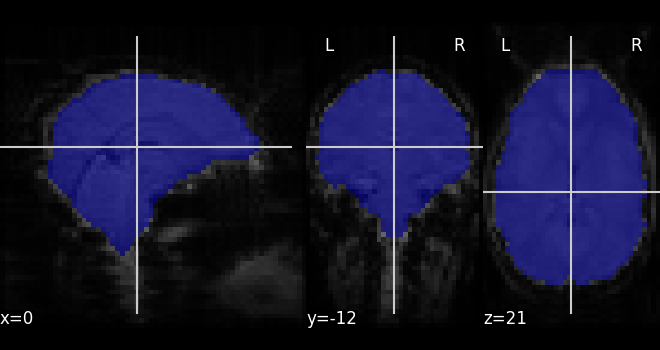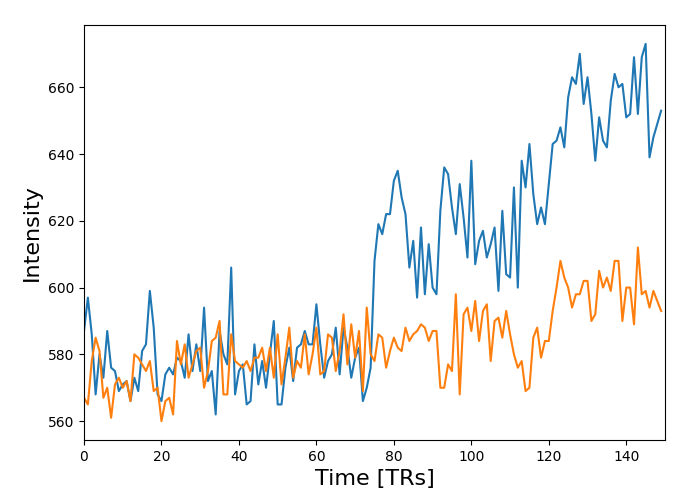# NeuroImaging volumes visualization#

Simple example to show Nifti data visualization.

## Fetch data#

```from nilearn import datasets

# By default 2nd subject will be fetched
haxby_dataset = datasets.fetch_haxby()

# print basic information on the dataset
print(
f"First anatomical nifti image (3D) located is at: {haxby_dataset.anat}"
)
print(
f"First functional nifti image (4D) is located at: {haxby_dataset.func}"
)
```
```First anatomical nifti image (3D) located is at: /home/runner/work/nilearn/nilearn/nilearn_data/haxby2001/subj2/anat.nii.gz
First functional nifti image (4D) is located at: /home/runner/work/nilearn/nilearn/nilearn_data/haxby2001/subj2/bold.nii.gz
```

## Visualization#

```from nilearn.image.image import mean_img

# Compute the mean EPI: we do the mean along the axis 3, which is time
func_filename = haxby_dataset.func
mean_haxby = mean_img(func_filename)

from nilearn.plotting import plot_epi, show

plot_epi(mean_haxby, colorbar=True, cbar_tick_format="%i")
``````<nilearn.plotting.displays._slicers.OrthoSlicer object at 0x7fd0eca04460>
```

Simple computation of a mask from the fMRI data

```from nilearn.masking import compute_epi_mask

# Visualize it as an ROI
from nilearn.plotting import plot_roi

``````<nilearn.plotting.displays._slicers.OrthoSlicer object at 0x7fd0ed022160>
```

## Applying the mask to extract the corresponding time series#

```from nilearn.masking import apply_mask

# masked_data shape is (timepoints, voxels). We can plot the first 150
# timepoints from two voxels

# And now plot a few of these
import matplotlib.pyplot as plt

plt.figure(figsize=(7, 5))
plt.xlabel("Time [TRs]", fontsize=16)
plt.ylabel("Intensity", fontsize=16)
plt.xlim(0, 150)